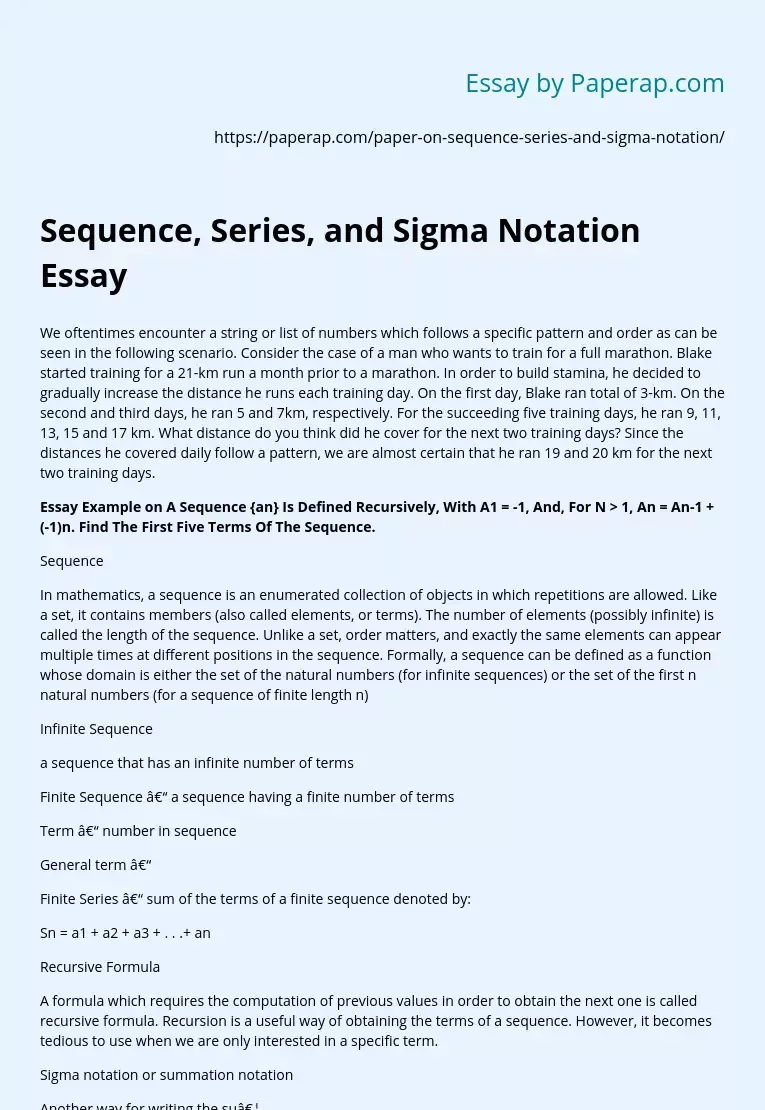# Sequence, Series, and Sigma Notation Essay

We oftentimes encounter a string or list of numbers which follows a specific pattern and order as can be seen in the following scenario. Consider the case of a man who wants to train for a full marathon. Blake started training for a 21-km run a month prior to a marathon. In order to build stamina, he decided to gradually increase the distance he runs each training day. On the first day, Blake ran total of 3-km. On the second and third days, he ran 5 and 7km, respectively.

For the succeeding five training days, he ran 9, 11, 13, 15 and 17 km. What distance do you think did he cover for the next two training days? Since the distances he covered daily follow a pattern, we are almost certain that he ran 19 and 20 km for the next two training days.

In mathematics, a sequence is an enumerated collection of objects in which repetitions are allowed. Like a set, it contains members (also called elements, or terms).

The number of elements (possibly infinite) is called the length of the sequence. Unlike a set, order matters, and exactly the same elements can appear multiple times at different positions in the sequence. Formally, a sequence can be defined as a function whose domain is either the set of the natural numbers (for infinite sequences) or the set of the first n natural numbers (for a sequence of finite length n)

Infinite Sequence a sequence that has an infinite number of terms Finite Sequence – a sequence having a finite number of terms Term – number in sequence General term – Finite Series – sum of the terms of a finite sequence denoted by: Sn = a1 + a2 + a3 + .

Get quality help nowBella HamiltonVerified

Proficient in: Logic5 (234)

“ Very organized ,I enjoyed and Loved every bit of our professional interaction ”+84 relevant experts are online

. .+ an Recursive Formula A formula which requires the computation of previous values in order to obtain the next one is called recursive formula. Recursion is a useful way of obtaining the terms of a sequence. However, it becomes tedious to use when we are only interested in a specific term. Sigma notation or summation notation Another way for writing the su…